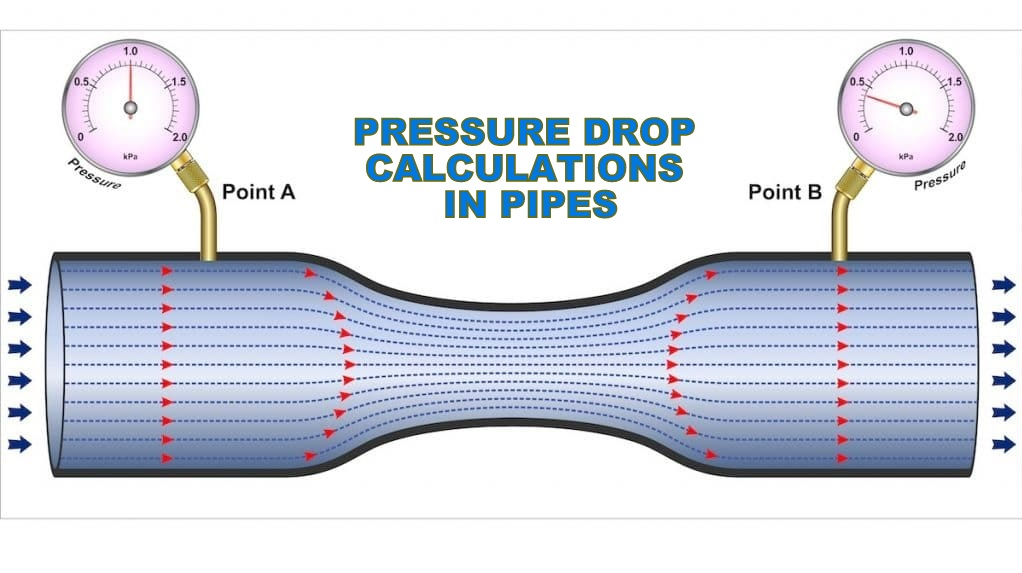# Pressure Drop or Head Loss, Occurs in all Piping SystemsPressure drop or head loss, occurs in all piping systems because of elevation changes, turbulence caused by abrupt changes in direction, and friction within the pipe and fittings. The most common methods used to determine the head loss in fiberglass pipe are Hazen-Williams, Manning and Darcy-Weisbach equations. The suitability of each method depends on the type of flow (gravity or pumped) and the level of accuracy required. Due to the smooth inside surface and the resistance to corrosion, ADPF fiber glass pipes have a relatively low head loss as compared to other material pipes. See Pressure loss versus flow rate curves.

Hazen-Williams Equation :-
The Hazen-Williams Equation is applicable to water pipes under conditions of full turbulent flow. It has gained wide acceptance in the water and wastewater industries because of its simplicity.

where,
v = velocity, m/s
C = Hazen-Williams Coefficient
R = Hydraulic mean radius, m
Hazen-William coefficient, C for ADPF fiber glass pipe is taken as 150.

Manning Equation :-
The Manning equation typically solves gravity flow problems where the pipe is only partially full and is
under the influence of an elevation head only.where,
v = velocity, m/s
n = Manning’s Coefficient
R = Hydraulic mean radius, m
Manning’s Coefficient, n for ADPF fiber glass pipe is taken as 0.01.

Darcy-Weisbach Equation :-
It states that pressure drop is proportional to the square of the velocity and the length of the pipe. This
equation is valid for all fluids in both laminar and turbulent flow. The disadvantage is that the Darcy-
Weisbach friction factor is a variable.

where,
g = Gravity constant, 9.81 m/s²
v = Velocity, m/s
D = Inside diameter, m
f = Friction factor
L = Length of the pipe, m

The well known Reynolds number equation is used to characterize the fluid flow.

Re = v.D / ν

where
ν= Kinematic viscosity, m²/s
The table given below determines the type of flow of fluid from the Reynolds number.

If the flow is Laminar, f = 64 / Re
If the flow is Turbulent, the friction factor can be determined from the Moody diagram found in most fluid mechanics texts or calculated from the Colebrook equation.

where,
ε = Long term absolute roughness.
Re = Reynolds number
The Colebrook friction factor for ADPF fiber glass pipe is determined as 0.04 mm including head losses
over joints.

Pressure drop in fittings....
Head Loss in Fittings is frequently expressed as the equivalent length of pipe that is added to the
straight run of pipe as shown below. This approach is used most often with the Hazen-Williams or
Manning’s equations. The approach does not consider turbulence and subsequent losses created by different velocities.

EQUIVALENT LENGTH IN METERS :-

When more accuracy is required, head loss in fittings can be determined using loss coefficients (Kfactors) for each type of fittings. In this approach K-factor is multiplied by the velocity head of the fluid flow.

where,
V = Velocity of flow, m/s

K-FACTOR FOR GRP FITTINGS :-

Surge pressure (Water Hammer)....
Pressure surge or Internal shock, known commonly as water hammer, results from abrupt change of velocity within the system. Under certain conditions, these shock forces can reach magnitude sufficient to rupture or collapse a piping system, regardless of the material of construction. The transient pressure is the rapidly moving wave which increases and decreases the pressure in the system depending on the source of the transient and direction of wave travel. Rapid valve closure can result in the build-up of shock waves due to the conversion of kinetic energy of the moving fluid to potential energy which must be accommodated. These pressure waves will travel throughout the piping system and can cause damage far away from the wave source.

The magnitude of the water hammer depends on,
• Fluid properties
• Velocity of flow
• Modulus of Elasticity of the pipe material
• Length of the pipe line and
• Speed in which the momentum of the fluid changes

The low modulus of elasticity of fiberglass contributes to a self dampening effect as the pressure wave
travels through the piping system. The magnitude of pressure wave in a metallic piping system is much higher due to the higher modulus of elasticity of these materials. In addition to rapid valve closure or opening, sudden air release and pump start up or shut down can create water hammer.

Talbot formula gives:

where,
a=Wave velocity (ft/s)
P = Surge Pressure (psi)
v = Change in flow velocity (ft/s)
w = Density of fluid (lb/ft³)
SG = Specific gravity of fluid
K = Bulk modulus of fluid (psi)
E = Hoop modulus of elasticity (psi)
d = Inside diameter of pipe (inch)
t = Pipe wall thickness (inch)
g = Acceleration due to gravity (ft/s²)

Good design practice usually prevents water hammer in most systems. Installation of valves which cannot open or close rapidly is one simple precaution. In addition, pumps should never be started into empty discharge lines unless slow opening mechanically actuated valves can increase the flow rate gradually. Check valves on pumps should close as quickly as possible to minimize the velocity of fluid flowing back. In some cases, thoroughly anchoring the piping system may mitigate this problem. In other cases, mechanical valve operators, accumulators, or feedback loops around pumps may have to be used to remove the source of water hammer.